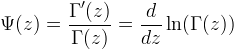Computes the digamma function.

Namespace:  Meta.Numerics.Functions
Assembly:  Meta.Numerics (in Meta.Numerics.dll) Version: 4.1.4Syntax
```public static double Psi(
double x
)```

#### Parameters

x
Type: SystemDouble
The argument.

#### Return Value

Type: Double
The value of ψ(x).Remarks

The psi function, also called the digamma function, is the logrithmic derivative of the Γ function.Because it is defined as a logarithmic derivative, the digamma function does not overflow Double even for arguments for which Gamma(Double) does.

To evaluate the psi function for complex arguments, use Psi(Complex).See Also